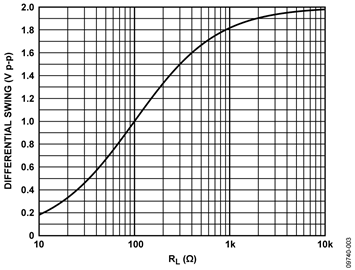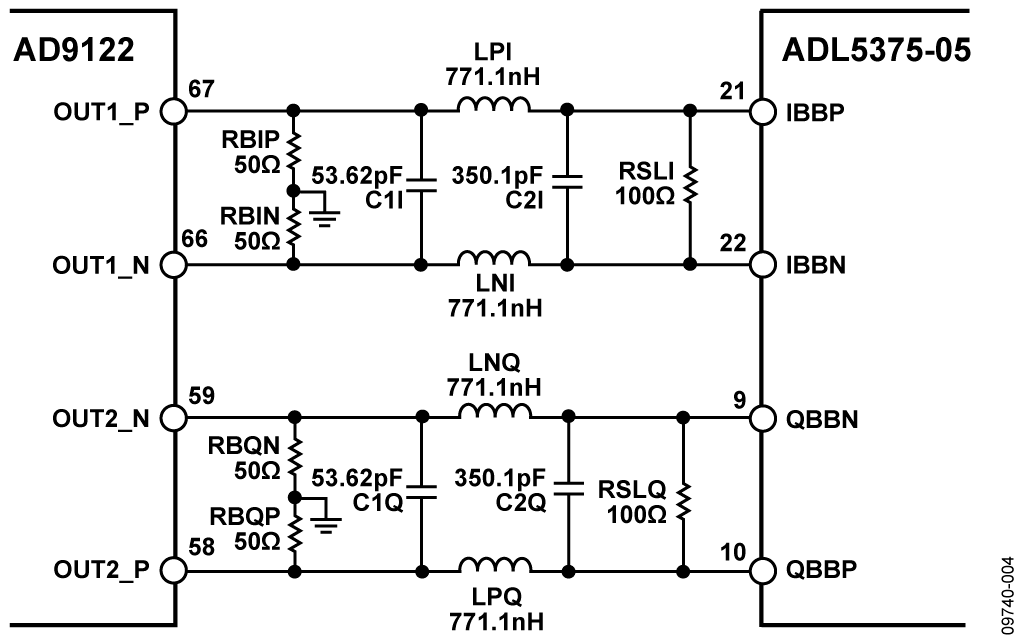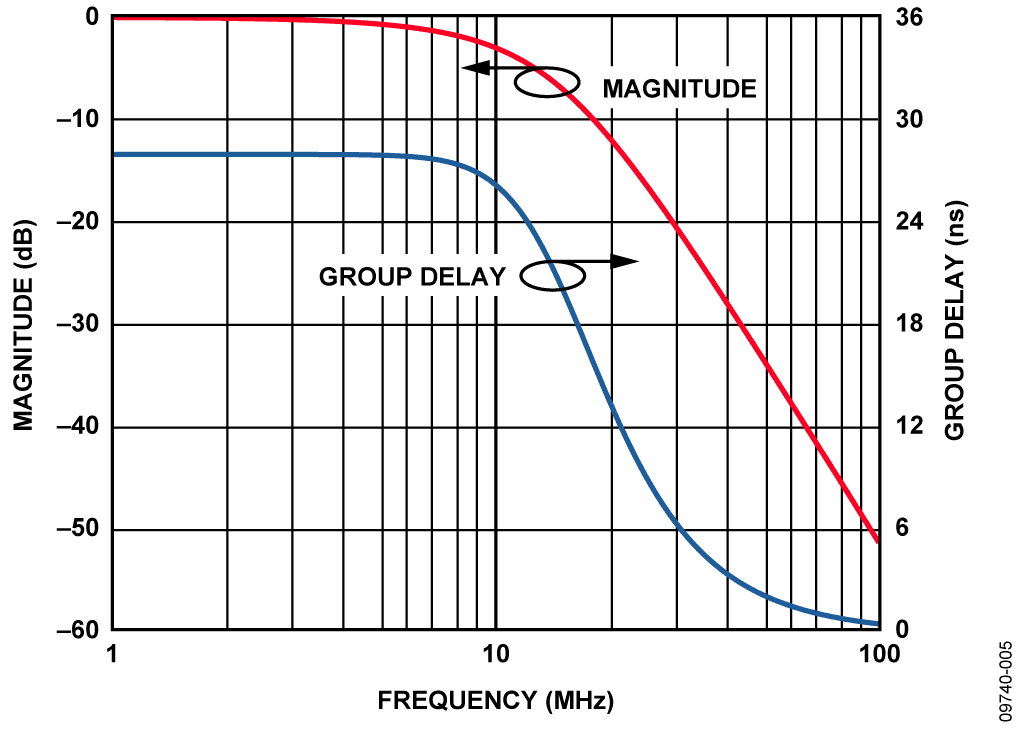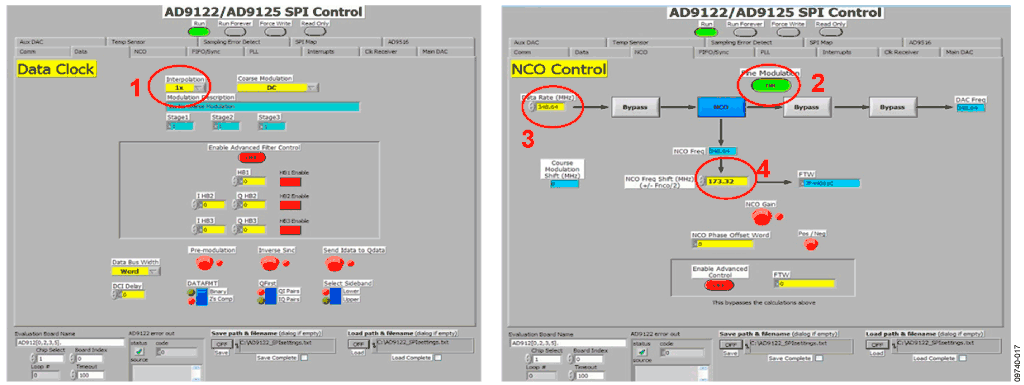Evaluation Hardware \$495.00

## Design Resources

### Design & Integration Files

• Schematic
• Bill of Materials
• Gerber Files
• Allegro Files
• Assembly Drawing

### Evaluation Hardware

Part Numbers with "Z" indicate RoHS Compliance. Boards checked are needed to evaluate this circuit

• AD9122-M5375-EBZ (\$495.00) Circuit Evaluation board designed to evaluate CN0205.

## Features & Benefits

• High speed DAC to IQ modulator interface
• Requires zero IF frequencys
• Dual DAC provides common biasing for level shifting

## Circuit Function & Benefits

This circuit provides a simple and flexible interface between the AD9122 dual high speed TxDAC digital-to-analog converter and the ADL5375-05 broadband I/Q modulator. Because the DAC outputs and ADL5375-05 I/Q modulator inputs share a common bias level of 0.5 V, there is no need for any active or passive level shifting circuitry. The dc coupled interface facilitates I/Q modulator local oscillator (LO) leakage compensation by the DAC.

The 1.2 GSPS AD9122 DAC sampling rate and the wide bandwidth of the ADL5375-05 modulator I and Q inputs ensure that both zero-IF (ZIF) or complex-IF (CIF) architectures can be supported. In addition to filtering Nyquist images, the baseband filter provides excellent rejection of both differential-mode and common-mode DAC spurs.

## Circuit Description

The circuit and board shown in Figure 1 and Figure2 utilize the AD9122 TxDAC and the ADL5375-05 wideband transmit modulator. Signal biasing and scaling in the interface circuit is controlled by the four ground-referenced resistors (RBIP, RBIN, RBQP, RBQN) and the two shunt resistors (RSLI,RSLQ), respectively.Figure 1. Interface Between the AD9122 and ADL5375-05 with 50 Ω Resistors to Ground to Establish the 500 mV DC Bias for the ADL5375-05 Baseband Inputs (Simplified Schematic)

The DAC’s full-scale output current (IFS) is programmable from 10 mA to 30 mA. The nominal and default value is 20 mA. In this configuration, the DAC outputs swing from 0 mA to 20 mA across each of the four ground-referenced 50 Ω resistors (RB =RBIP = RBIN = RBQP = RBQN). This establishes the 500 mV dc bias level and a full-scale voltage swing of 2 V p-p differential on each output pair (with no load). This 2 V p-p voltage swing can be adjusted by the RL (RL = RSLI = RSLQ) shunt resistors without affecting the 500 mV bias level. The resulting differential peak-to-peak swing at the I/Q modulator input is given by the equationNote that the relatively high differential input impedance of the ADL5375 (typically >60 kΩ) can be ignored when calculating this signal level. Figure 3 shows the relationship between the peak-to-peak voltage swing and RL when 50 Ω bias-setting resistors are used.

The ADL5375-05 and AD9122 are well matched in terms of dynamic range and gain. As a result, there is no need for any active gain between the devices. The I/Q modulator drive level can be fine tuned as needed by adjusting the value of RL as described above. For most applications, a value of 100 Ω for RL is recommended. This results in a full-scale signal level of 1 V p-p (DAC output at 0 dBFS).Figure 3. Peak-to-Peak Differential Swing and the Swing Limiting Resistor (RL) with 50 Ω Bias-Setting Resistors

### Baseband Filtering

A filter must be inserted between the AD9122 and ADL5375 to remove Nyquist images, spurs, and broadband noise originating from the DAC. The filter should be placed between the dc bias setting resistors and the ac swing-limiting resistor. With this configuration, the dc bias setting resistors (RB in Figure 4) and the signal scaling resistors (RL in Figure 4) conveniently set the source and load resistances for the filter design.

Figure 4 shows a third-order Bessel low-pass filter with a −3 dB frequency of 10 MHz. Matching input and output impedances of the filter makes the filter design easy and results in better passband flatness, which allows wide bandwidth filter designs. In this example, the shunt resistor chosen is 100 Ω, producing an ac swing of 1 V p-p differential. The frequency response of this filter is shown in Figure 5.Figure 4. DAC Modulator Interface with 10 MHz Third-Order, Bessel FilterFigure 5. Frequency Response for DAC Modulator Interface with 10 MHz Third-Order Bessel Filter

### Filtering for Complex IF (CIF) Applications

Figure 6 shows the frequency response of the ADL5375 baseband I and Q inputs. Because this device has a wide and flat frequency response (−3 dB point = 750 MHz), it is well suited to complex IF (CIF) applications where the output signal from the DAC has been digitally upconverted. In CIF applications, a lowpass Nyquist filter is still desirable, primarily because the dc bias level can be preserved from the DAC output to the modulator input.

The filter topology shown in Figure 7 is a 5th order Butterworth filter with a 300 MHz corner frequency and is the recommended filter topology. A purely differential filter can reject differential-mode images, spurs, and noise from the DAC. Using two capacitors with their common connection grounded (C2 and C4 in Figure 7) diverts some of the common-mode current to ground and results in better common-mode rejection of high-frequency signals than would be obtained with a purely differential filter.

The simulated and measured responses of this filter are shown in Figure 8 and Figure 9. The measured flatness is ±0.6 dB from dc to 250 MHz and ±0.4 dB from 125 MHz to 250 MHz. This data was taken with the AD9122 inverse sinc function on. In this configuration, Figure 10 shows the common-mode rejection performance of the 2 × FDAC common-mode spur vs. common-mode frequency with and without IF filter shown in Figure 7.Figure 7. Recommended DAC Modulator Interface topology with Fc =300 MHz Fifth-Order, Butterworth FilterFigure 8. Frequency Response for DAC Modulator Interface with 300 MHz Fifth-Order Butterworth Filter (Simulated)Figure 9. Measured Frequency Response for DAC Modulator Interface with 300 MHz Fifth-Order Butterworth FilterFigure 10. Measured Common-Mode Rejection Performance at ADL5375-05 RF output with Filter and without Filter

In addition to the bias-setting and signal scaling resistors, the power level at the output of the ADL5375 is a function of the DAC’s digital backoff level (dBFS), the signal’s peak-to-average ratio, the DAC’s full-scale current, the insertion loss of the Nyquist filter, and I/Q modulator’s voltage gain. The spreadsheet shown in Figure 11 can be used to make this calculation.

### Level Shifting to Drive the ADL5375-15

The ADL5375-15 requires a dc bias level of 1500 mV. Other than the difference in bias levels, the ADL5375-05 and ADL5375-15 are identical. To drive the ADL5375-15 from the AD9122, either a passive or active level-shifting network must be used. The passive level shifting network shown in Figure 12 uses four series resistors along with four pull-up resistors to achieve a bias level of 1500 mV at the ADL5375-15 input. This passive level shifting network introduces a loss of approximately 2 dB in the signal level.

An active level shifting circuit would use a dual-differential amplifier, such as ADA4938-2, where placing 1500 mV on the VOCM pin sets the output dc bias level. In this approach, however, the interface bandwidth is limited by the op amp.

As previously mentioned, it is necessary to put a filter between AD9122 and ADL5375-15. The LC filter can be located anywhere between the DAC termination resistors (R1 in Figure 13) and the ac swing-limiting resistor (R4 in Figure 13). However, the circuit in Figure 13 allows flexibility in the design of the level shifting circuit with low loss by R2 and a high driving level to modulator. It also allows a matched filter at source and load impedance. Figure 13 is the recommended passive levelshifting network with filter.

The differential source impedance and load impedance of the filter are

2 × (R1 + R2) and
2 × {R3||(R4/2)}, respectively.

The single-ended impedance seen by DAC is

R1||{R2+R3||(R4/2)}.

R4 acts as the ac load to the DAC. The differential ac swing at DAC output is

2 × IFS × R1||{R2+R3||(R4/2)},

and the differential ac swing at the modulator input is

2 × {R3||(R4/2)}÷{R2+(R3||(R4/2)}

multiplied by the differential ac swing at DAC output.

The LC filter should be placed close to the DAC to allow short return current path. The 5 V bias supply (V1) should be close to the modulator because it is shared with the modulator. For the case when R1, R2, R3, and R4 are 34 Ω, 218 Ω, 760 Ω, and 750 Ω, respectively, the 500 mV dc bias at the AD9122 DAC output is matched to the 1500 mV dc bias at ADL5375-15. Actually, it is not necessary to be 500 mV at point A of Figure 13, but it will give flexibility in the ac swing level without exceeding the compliance voltage of the DAC output. The DAC load is 31.7 Ω. The input and output impedance of the filter are 504 Ω and 502 Ω. The attenuation by R2, which is the voltage drop by R2 between the DAC output and modulator input, is set by the combination of R2 and R3||(R4/2),which is about 5.4 dB.

To calculate dc bias level and ac swing level at the A and B points (Figure 13), attenuation by R2, and source/load impedances of the filter, the spreadsheet below can be used. This can be downloaded at the following URL: www.analog.com/CN0205-LevelShifter.

### Layout Recommendations

Special care should be taken in the layout of the DAC/modulator interface. Here are some recommendations. Figure 15 shows a top-level layout, which follows these recommendations:

• Keep all I/Q differential trace lengths well matched.
• Place filter termination resistor as close as possible to modulator input.
• Place DAC output 50 Ω resistors as close as possible to DAC.
• Thicken trace widths through the filter network to reduce signal loss.
• Place vias around all DAC output traces, filter networks, modulator output traces, and LO input traces.
• Route LO and modulator outputs on different layers or at 90° angle to each other to prevent coupling.

Further insight to proper layout can be found by examining the AD9122-M5375-EBZ layout files in the design support package www.analog.com/CN0205-DesignSupport.

## Common Variations

The interface can also be adapted to the AD8345/AD8349 low current modulators, with some adjustment to the bias level by properly selecting the DAC termination resistors.

## Circuit Evaluation & Test

The following section describes details of performing the common-mode test (results shown in Figure 10). The test setup is flexible and allows other measurements shown in this circuit note to be performed.

### Equipment Needed (Equivalents Can be Substituted)

• DPG : ADI Digital Pattern Generator
• Signal Generator for clock: Agilent E4437B
• Signal generator for LO: Agilent 8665B
• Spectrum analyzer: Agilent E4440A
• Power supply: Agilent E3631A

### Setup and Test

1. Connect the setup and measurement system shown in Figure 16.
2. Set the power supply to +5 V.
3. Set the signal generator for FDAC to 368.64 MHz @ 5 dBm, and the signal generator for LO to 2140 MHz @ 0 dBm.
4. Turn on the power supply and signal generators. Set the spectrum analyzer at 2 × FDAC MHz, 1 MHz span.
5. Set up the AD9122 through USB at AD9122/AD9125 SPI control software as shown in Figure 17 and run. Refer to the AD9122 Evaluation Board Quick Start Guide in www.analog.com-CN0205-DesignSupport.
• Interpolation ("1" in Figure 17) : 1×
• Fine modulation ("2" in Figure 17) : ON
• Data rate ("3" in Figure 17) : same as FDAC frequency
• NCO frequency ("4" in Figure 17) : 173.32 MHz
6. Set up DPG (refer to AD9122 Evaluation Board Quick Start Guide)
• Make sure DCO frequency ("1" in Figure 18) is close to FDAC frequency.
• Set sample rate ("2" in Figure 18) same as FDAC frequency and 1 MHz at desired frequency.
• Set "3" and "4 "as shown in Figure 18.
• Download I and Q vector by clicking buttons at "1" in Figure 18.
7. Measure common-mode noise levels at 2 × FDAC
8. Change frequency of signal generator for FDAC, change “Data Rate” mentioned in (5), and “Sample Rate” mentioned in (6)
9. Measure common-mode noise levels at 2 × FDAC (NEW)
10. Repeat (8), (9)Figure 17. SPI Control User Interface Setup for Data Clock and NCO Control